註冊 忘記密碼
 .jqx-menu-icon{float: left; margin-right: 5px; width:16px; }
 CTIMES / 文章 /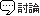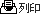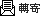不插電學AI：快樂學習人工智慧 【作者： 高煥堂】   2018年12月25日 星期二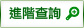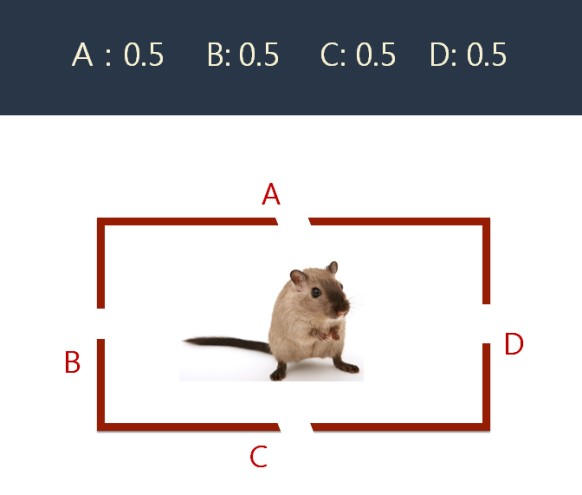圖1 : 不知道洞外是否有貓圖2 : 探索A洞（圖片引自妮圖網）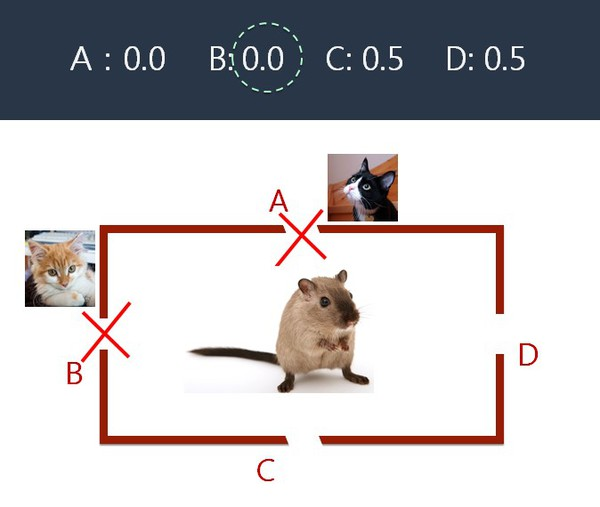圖3 : 也就記住了B洞外有貓圖4 : 記住了C洞外沒有貓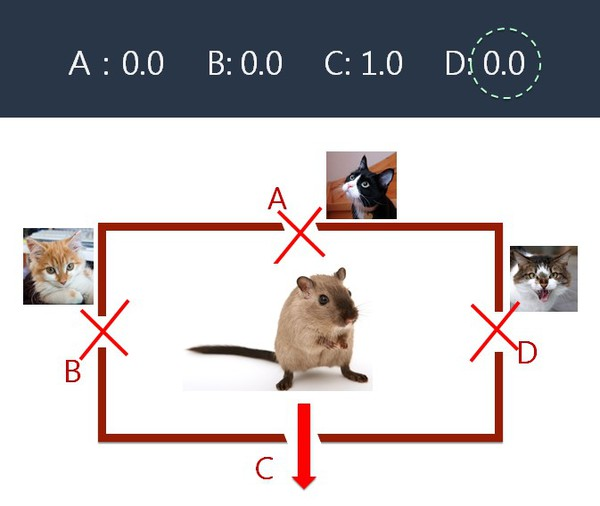圖5 : 又記住了D洞外有貓圖6 : 寫下了牠探索時的選擇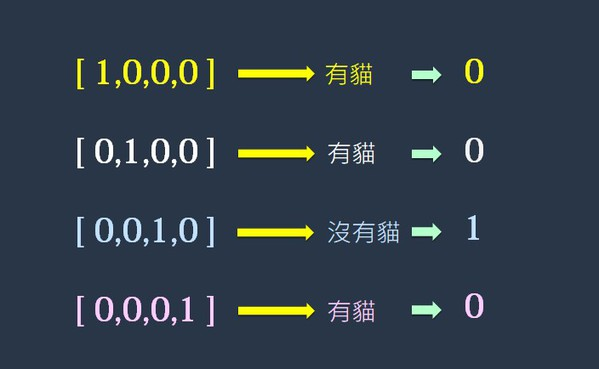圖7 : 把探索的結果也寫下來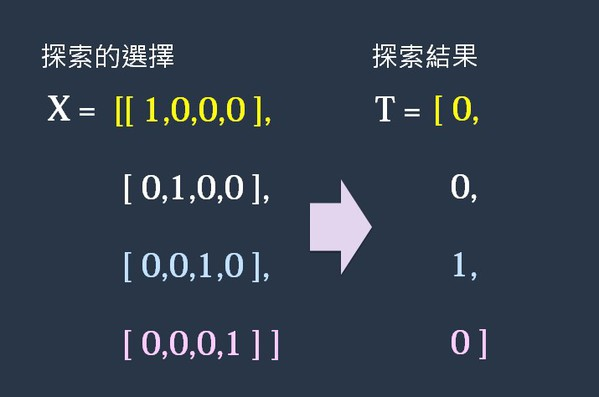圖8 : 拿X[]和T[]陣列來表示兩項資料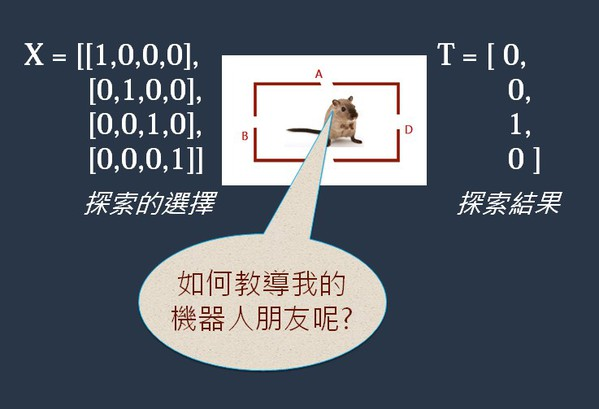圖9 : 把智慧表示出來

y = x1*0.5 + x2*0.5 + x3*0.5 + x4*0.5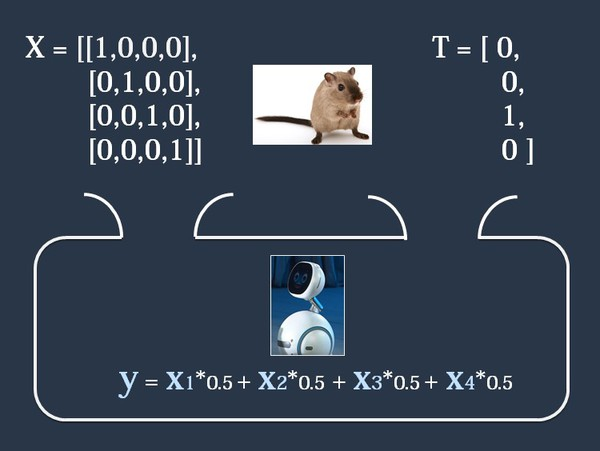圖10 : 把數學式放入機器人腦海裡

[x1, x2, x3, x4] = [1, 0, 0, 0]圖11 : 帶入X[]資料，展開訓練圖12 : 估算第1次探索的成功信心度（即y值）圖13 : 比較一下估算值與實際值圖14 : 修正數學式的參數（權重）圖15 : 智慧成長了

y = x1*0.0 + x2*0.5 + x3*0.5 + x4*0.5

[x1, x2, x3, x4] = [1, 0, 0, 0]

y = x1*0.0 + x2*0.5 + x3*0.5 + x4*0.5

= 1 * 0.0 + 0 * 0.5 + 0 * 0.5 + 0 * 0.5

= 0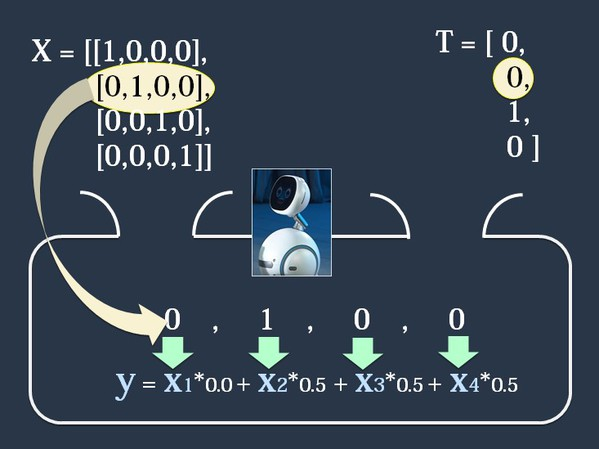圖16 : 帶入X[]資料，展開第2筆的訓練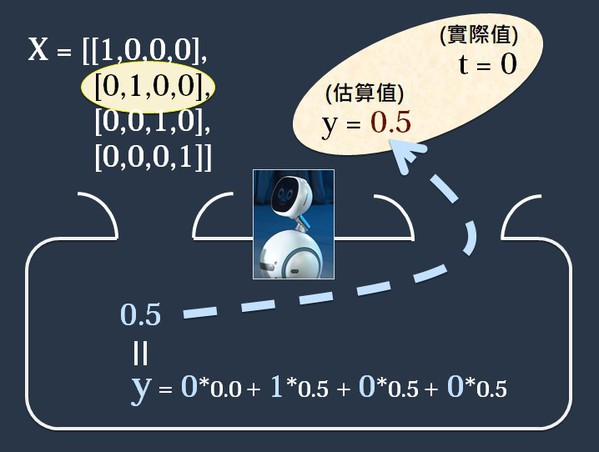圖17 : 比較一下估算值與實際值圖18 : 修正數學式的權重圖19 : 智慧繼續成長了

y = x1*0.0 + x2*0.0 + x3*0.5 + x4*0.5

[x1, x2, x3, x4] = [0, 1, 0, 0]

y = x1*0.0 + x2*0.0 + x3*0.5 + x4*0.5

= 0 * 0.0 + 1 * 0.0 + 0 * 0.5 + 0 * 0.5 = 0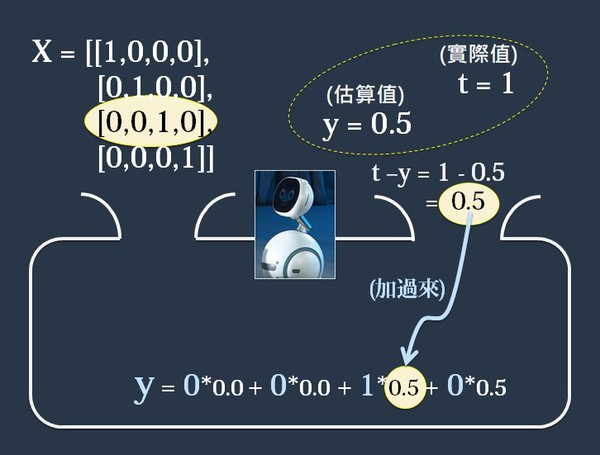圖20 : 修正數學式的權重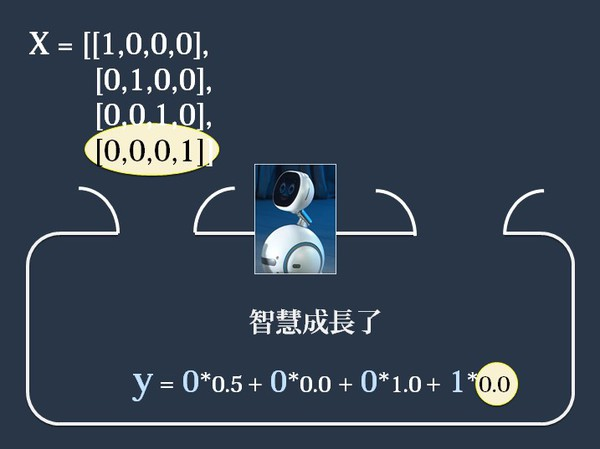圖21 : 經過4筆資料的訓練

W = [w1, w2, w3, w4] = [ 0.0, 0.0, 1.0, 0.0]

Step-1

y = x1*w1 + x2*w2 +x3*w3 + x4*w4

= 1*0.0 + 0*0.0 + 0*1.0 + 0*0.0 = 0

Step-2

y = x1*w1 + x2*w2 +x3*w3 + x4*w4

= 0*0.0 + 1*0.0 + 0*1.0 + 0*0.0　= 0

Step-3

y = x1*w1 + x2*w2 +x3*w3 + x4*w4

= 0*0.0 + 0*0.0 + 1*1.0 + 0*0.0 = 1.0

 相關文章
 ‧ 實現物聯網與雲端運算的新型記憶體技術 ‧ 仿真和原型難度遽增 Xilinx催生世界最大FPGA ‧ 新一代記憶體發威 MRAM開啟下一波儲存浪潮 ‧ 使機器學習推論滿足實際效能需求 ‧ 機器學習實現AI與EDA的完美匹配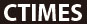︱ Copyright ©1999-2019 遠播資訊股份有限公司版權所有 Powered by O3 地址:台北市中山北路三段29號11樓 / 電話 (02)2585-5526 / E-Mail: webmaster@ctimes.com.tw